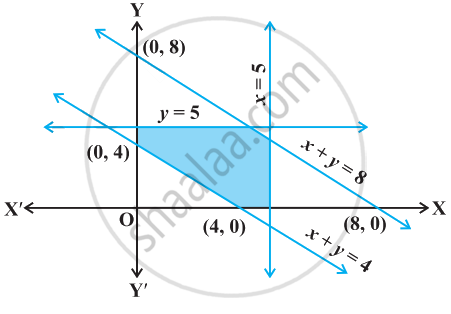# Find the linear inequalities for which the shaded region in the given figure is the solution set. - Mathematics

Sum

Find the linear inequalities for which the shaded region in the given figure is the solution set.#### Solution

Considering x + y = 8, the shaded region and the origin both are on the same side of the graph of the line and (0, 0) satisfy the constraint x + y ≤ 8.

Considering x + y = 4, the origin is on the opposite side of the shaded region and (0, 0), Hence, doesn’t satisfy the constraint x + y ≥ 4. Therefore required constraint is x + y ≥ 4.

We see that, The shaded region is in the first quadrant i.e. x ≥ 0 and y ≥ 0, Also, shades region is below the line y = 5 and left to the line x = 5.

⇒ y ≤ 5 and x ≥ 5

Hence, the linear inequalities are x + y ≤ 8,  x + y ≥ 4, x ≥ 0, y ≥ 0, x ≤ 5, y ≤ 5.

Concept: Solution of System of Linear Inequalities in Two Variables
Is there an error in this question or solution?

#### APPEARS IN

NCERT Mathematics Exemplar Class 11
Chapter 6 Linear Inequalities
Exercise | Q 15 | Page 108

Share